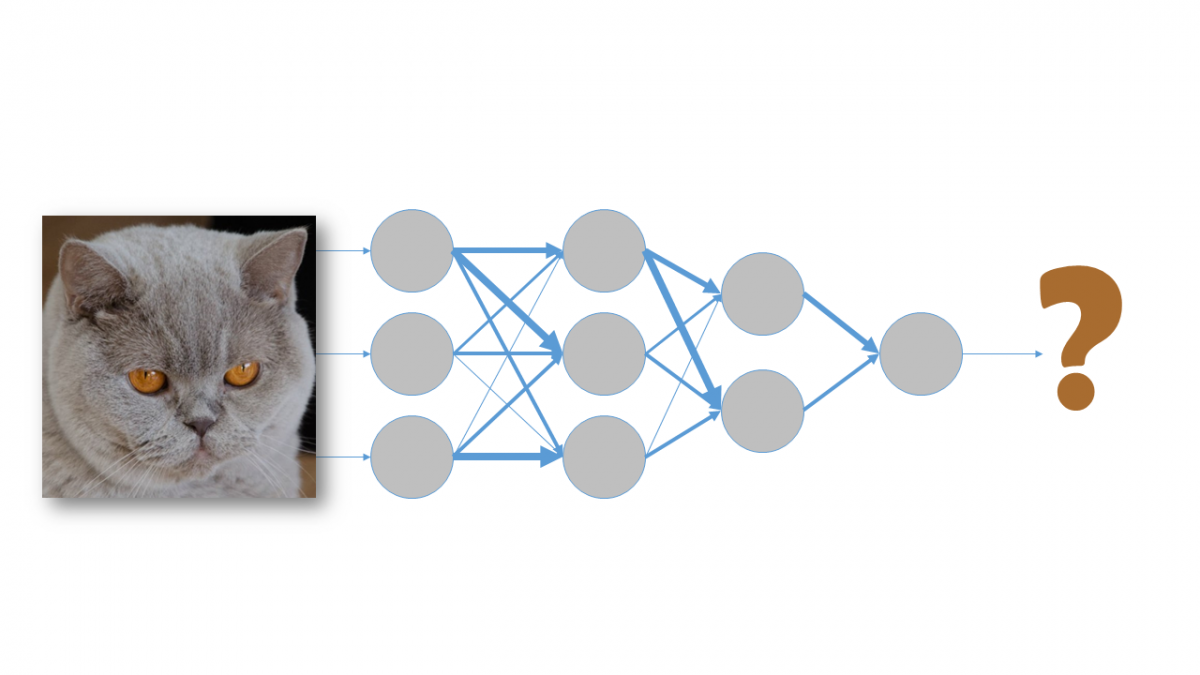# Training Artificial Neural Networks – Part IIThe boom in recent years of Machine Learning techniques in Artificial Intelligence prompted the brief introduction in our previous post to artificial neural networks and how to train them to obtain valuable information. In this post, we will analyse in a little more detail what is actually involved in training these types of networks.

## Backpropagation algorithm in artificial neural networks

The neural network training process is carried out using an algorithm called backpropagation, which we first mentioned in our blog post entitled What is Machine Learning? This term was coined because, to perform the training, it is necessary to:

1. Propagate input data through the network to obtain a prediction.
2. Calculate the error with respect to the expected output.
3. Work backwards through the network propagating this error in such a way that the neurons that were more significant in calculating the final result are more affected and are therefore modified more than the rest.

The gradient descent method is used to make this adjustment. However, this method fails when used with multilayered neural networks. In 1991, Hochreiter identified the two main problems that occurred when using this training technique:

• Gradient vanishing: When there are many layers, it is highly likely that, when reaching the lower layers of the network, the gradient value will be very close to zero, so the change in weights will be very small. This causes two problems: first, it takes a long time to train the network, and second, when the lower layers are not trained well enough, these errors spread through the entire network.
• Gradient explosion: In certain cases, the opposite may occur, causing the weight values to increase uncontrollably (or “explode”), preventing the network from being trained correctly.

To solve some of the problems identified in the gradient descent method, an activation function called ReLU (Rectified Linear Unit) was introduced in 2009.

The intrinsic characteristics of this function meant that models were trained much faster and neurons became more adept at extracting relevant information, resulting in neural networks with greater predictive power.

This is just one of the many reasons why the use of artificial neural networks and deep learning has become so popular today. In our next blog post, we’ll talk about this major revolution and how deep learning came about.

Oscar García-Olalla Olivera is a Data Scientist and R&D Engineer at Xeridia## The Importance of Data Preprocessing in Artificial Intelligence: Data Cleansing